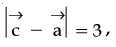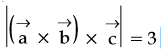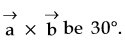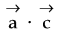Q

# Solve! - Vector Algebra - JEE Main

LetandLetbe a vector such thatand the angle betweenandthenis equal to:

• Option 1)• Option 2)• Option 3)• Option 4)112 Views

As we learnt in

Vector Triple Product -

- wherein

x and y are scalars.

and

Scalar Product of two vectors -

- wherein

is the angle between the vectors

Also,

Also,

So,

Option 1)This option is correct.

Option 2)This option is incorrect.

Option 3)This option is incorrect.

Option 4)This option is incorrect.

Exams
Articles
Questions